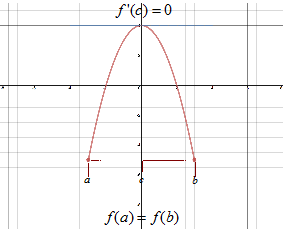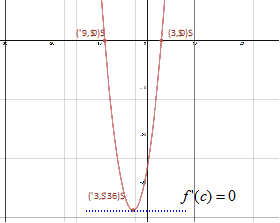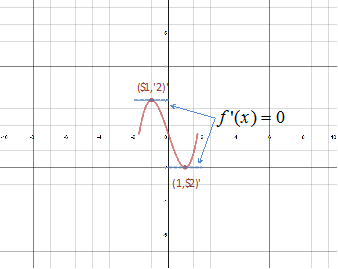# Rolle's Theorem

The Extreme Value Theorem states that on a closed interval a continuous function must have a minimum and maximum point. These extrema can occur in the interior or at the endpoints of the closed interval.

Rolle's Theorem states that under certain conditions an extreme value is guaranteed to lie in the interior of the closed interval.

ROLLE'S THEOREM

Conditions:

1. The function f must be continuous on the closed interval [a, b].

2. The function f must be differentiable on the open interval (a, b).

3. f(a) = f(b)

If these three conditions are met then there is at least one number in the open interval (a, b) such that the derivative of f is zero.Let's use this theorem in a couple examples.

Example 1: Find the two x-intercepts of the continuous function f(x) = x2 + 6x - 27 and show that f' (x) = 0 at some point between these two x-intercepts
 Step 1: Find the x-intercepts or zeros of the function. x2 + 6x - 27 = 0 Original (x + 9)(x - 3) = 0 Factor $x+9=0\to {x}{=}{-}{9}$    Set to zero & solve $x-3=0\to {x}{=}{3}$      Set to zero & solve Step 2: Find the first derivative of the function. $f\left(x\right)={x}^{2}+6x-27$ $f\prime \left(x\right)=2x+6$ Step 3: Solve for ${f}^{\prime }\left(x\right)=0$. 0 = 2x + 6 ${f}^{\prime }\left(x\right)=0$ -3 = x Solve Step 4: Compare x-intercepts to the value in Step 3 when ${f}^{\prime }\left(x\right)=0$. ${f}^{\prime }\left(-3\right)=0$ and -3 falls between -9 and 3, therefore there is a minimum point at (-3, -36).Example 2: The function f(x) = x3 - 3x is continuous and differentiable. Find the coordinates of all points in the interval such that f' (x) = 0.
 Step 1: Find the first derivative of the function. f(x) = x3 - 3x ${f}^{\prime }\left(x\right)=3{x}^{2}-3$ Step 2: Set the first derivative equal to zero and solve. ${f}^{\prime }\left(x\right)=3{x}^{2}-3$ First derivative 0 = 3x2 - 3      Set ${f}^{\prime }\left(x\right)=0$ 0 = 3(x2 - 1)   Factor out a 3 0 = 3(x - 1)(x + 1)    Factor the quad. $x-1=0\to x=1$    Solve for x $x+1=0\to x=-1$   Solve for x Step 3: Substitute the x-coordinates found in Step 2 into the function to determine the corresponding y-coordinates. $f\left(1\right)={\left(1\right)}^{3}-3\left(1\right)=-2$   f(1) = -2 $f\left(-1\right)={\left(-1\right)}^{3}-3\left(-1\right)=3$   f(-1) = 2 Step 4: Name the coordinates The coordinates of the two points where $f\prime \left(x\right)=0$ are (1, -2) and (-1, 2).Related Links: Math algebra The Mean Value Theorem Limits: Introduction and One-Sided Limits Calculus Topics

To link to this Rolle's Theorem page, copy the following code to your site: